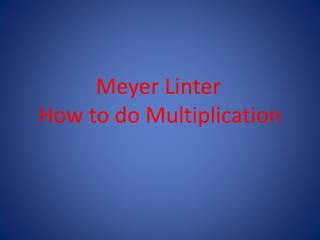DownloadDownload PresentationMeyer Linter How to do Multiplication

# Meyer Linter How to do Multiplication

Télécharger la présentation## Meyer Linter How to do Multiplication

- - - - - - - - - - - - - - - - - - - - - - - - - - - E N D - - - - - - - - - - - - - - - - - - - - - - - - - - -
##### Presentation Transcript

1. Meyer Linter How to do Multiplication

2. Multiplication(A better version of Addition) Multiplication is the same as repetitive addition. When you see 3 x 3 you can just add 3 + 3 + 3 and get 9. That’s the best thing about multiplication is when you get to big numbers you don’t have to do repetitive addition anymore you can just multiply.

3. Multiplication (Commutative Property) In multiplication it doesn’t matter if you have 3 x 4 or 4 x 3 because the answer will always be the same. Its unlike division where it DOES NOT matter in which order you have the two numbers. Your answer will always be the same, even when the numbers get really large.

4. Multiplication(Different ways of telling the outcome) • When you have an EVEN number multiplied by another EVEN number the outcome will be EVEN. • When you have an EVEN number multiplied by an ODD number the outcome will be EVEN. • When you have an ODD number multiplied by another ODD number the outcome will be ODD.

5. Multiplication(Different ways of learning) Everybody learns in a different way and here are some examples you guys can try: • Flash Cards • Multiplication Tables • Educational games • Working 1 on 1 with a partner

6. Multiplication(It takes time) Multiplication does not come easy for everybody and to understand and learn it you must practice. The only way you will get better at multiplication is practicing all kinds of problems at home and by yourself.